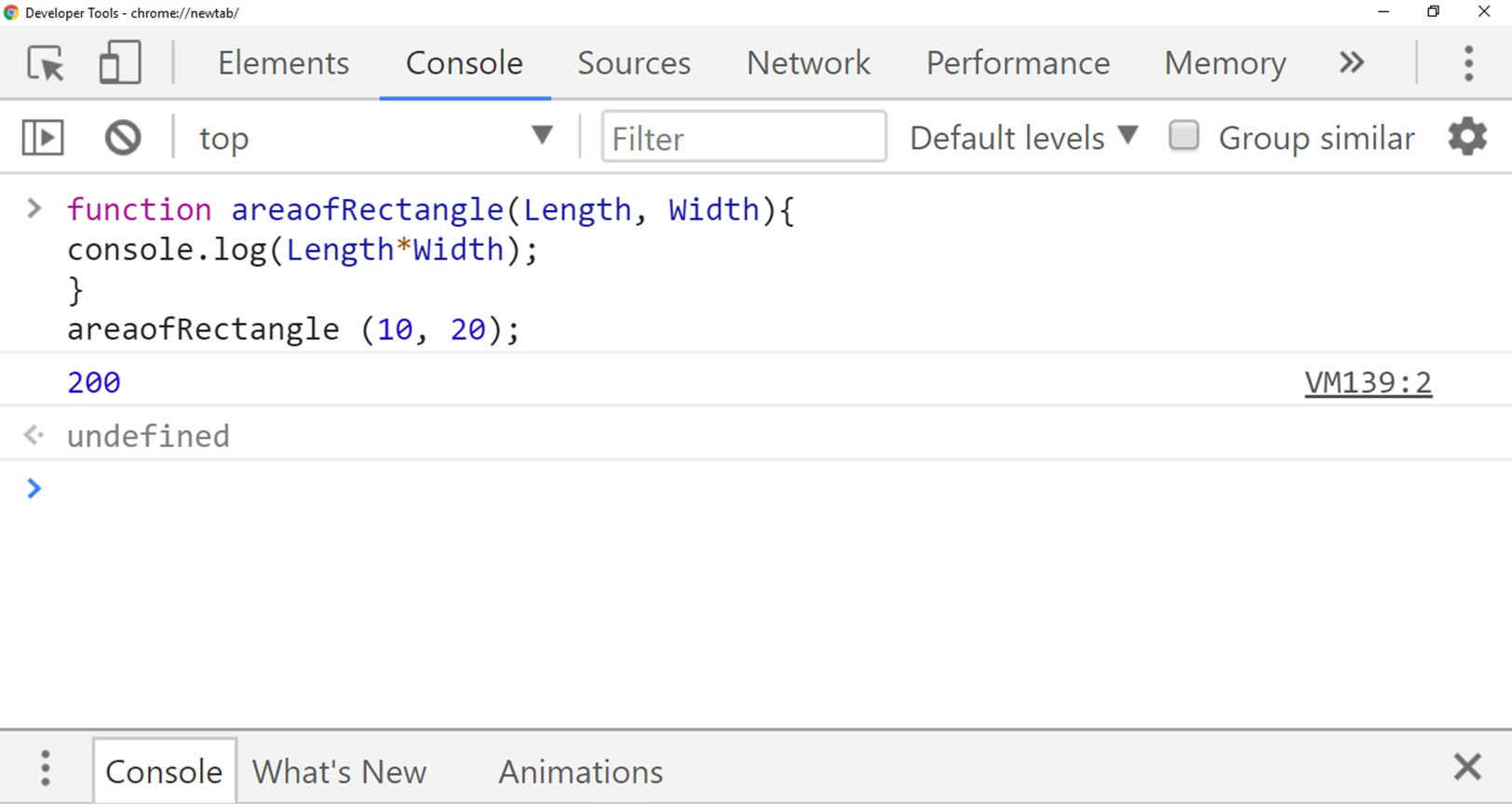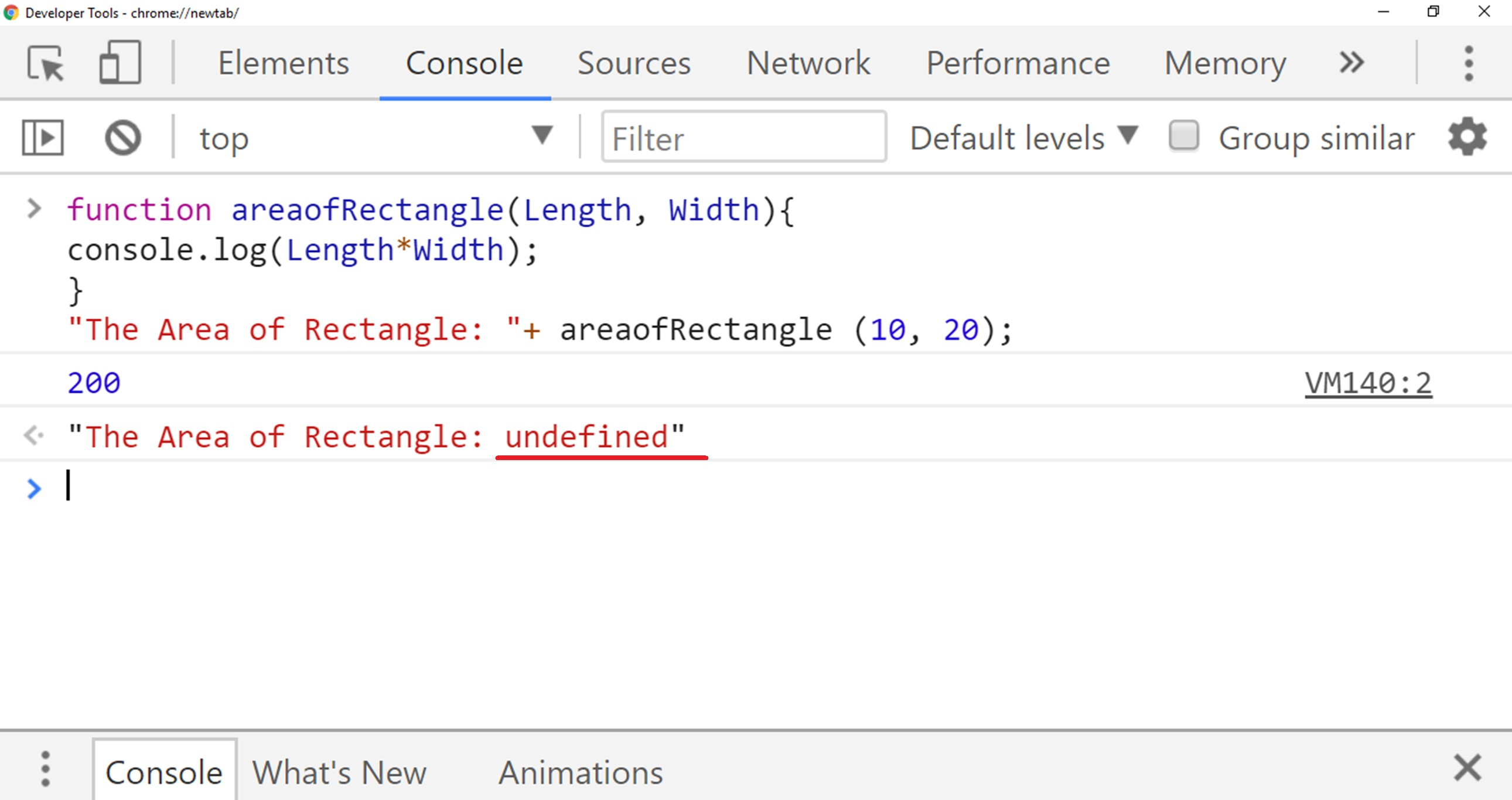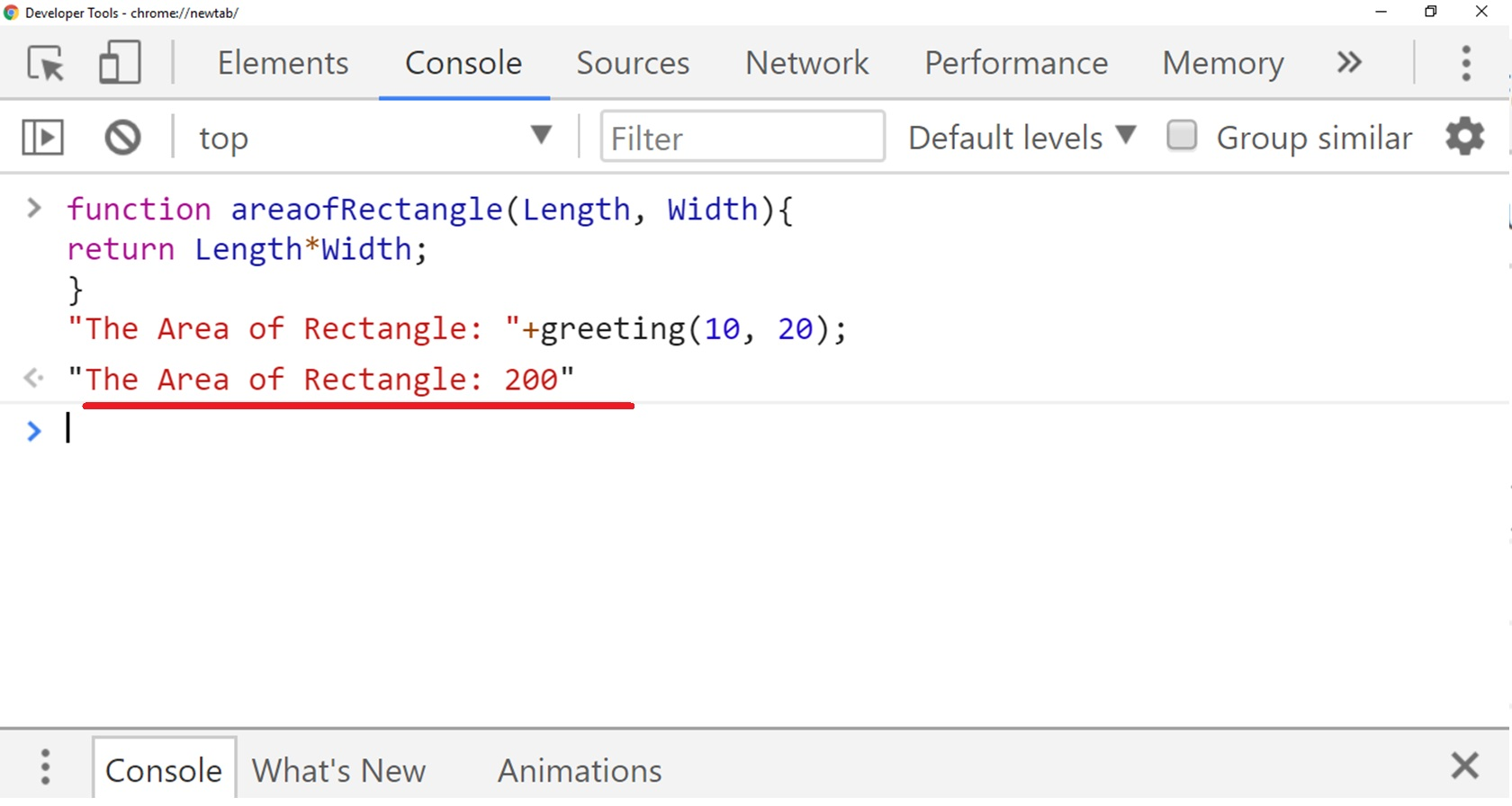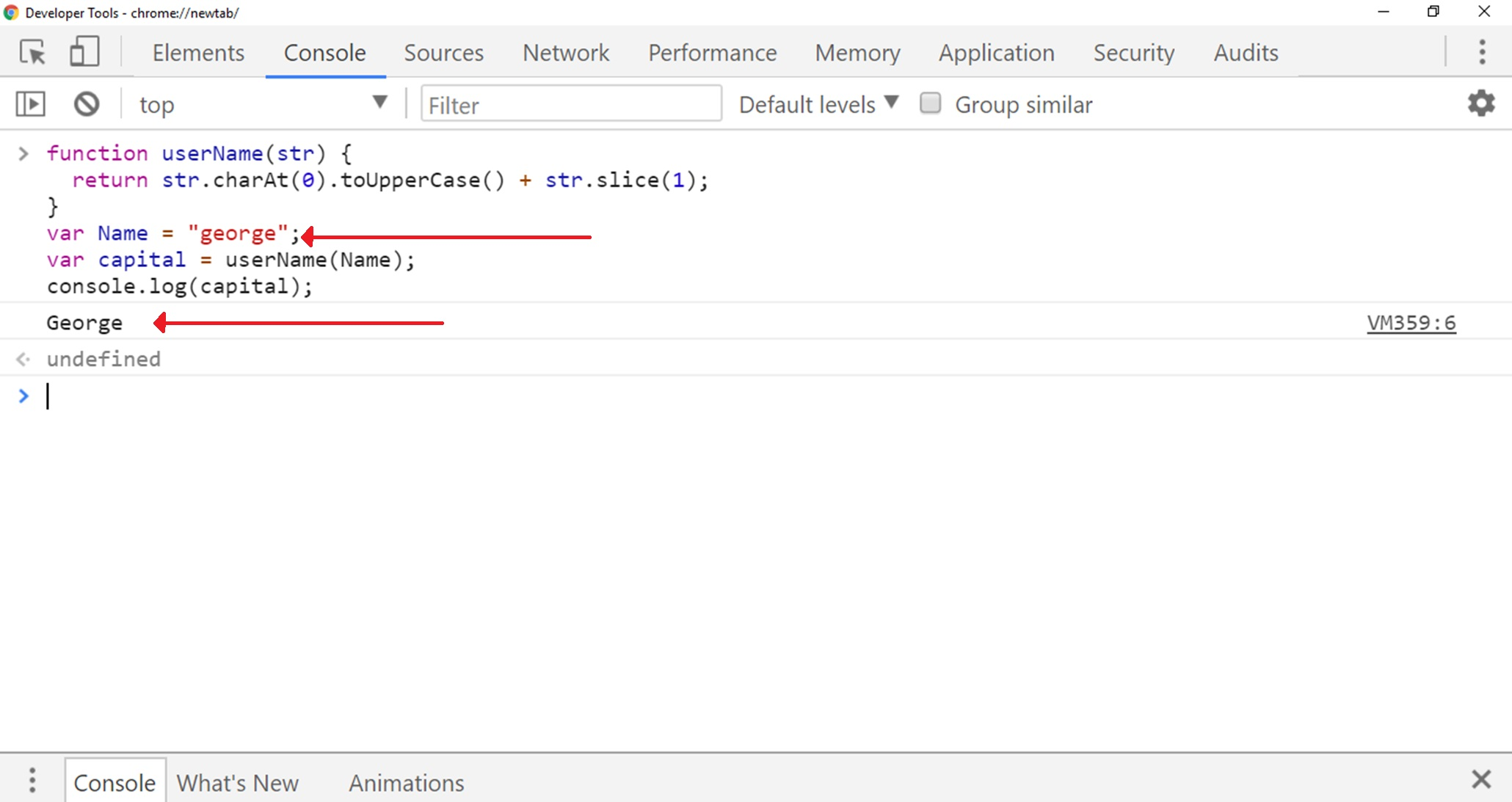# Lesson 15 Function Return Value

In this lesson, we are going to focus on last and another important concept of function that is return value.
So in this lesson, we are going to learn what the return keyword is and how do we write functions that use the return value.

## The Return Keyword

In the previous lessons, we used the function that has taken some inputs from users and after some calculation printed the result and they have not returned any significant value.
We use return keyword to send back value after completing function execution.
So return should need to be our last function statement and return stop the execution and send the output back to where from the function called.

### Syntax

``````function ourFunctionName(a, b) {
// Bunch of code to be executed
return a*b;
}
ourFunctionName(a, b);
``````

So I will show you what I mean if we have a function called areaofRectangle which we saw in the last lesson.
So in the previous lesson, all we did was print the value after multiplication of width and length.
And when I executed that code I got the area of rectangle printed on our console.

``````function areaofRectangle(Length, Width){
console.log(Length*Width);
}
areaofRectangle (10, 20);
``````

The following we will get if we run above code.In this case, we have got the result printed on our console because of console.log.
If we use return keyword instead of printing using console.log, then its mean that we can capture the value that is coming back out of the function.
If we do not return anything from the function then we got undefined in the result.So you can notice in above output that if we do not return anything from the function then we will get undefined in the result.
So now if we want to return back anything instead of printing in function, here is the code below:

``````function areaofRectangle(Length, Width){
return Length*Width;
}
"The Area of Rectangle: "+greeting(10, 20);
``````

So you can see that we have returned length*width from the function, here is the output below.So every function returns something and if we don’t explicitly tell it what to return then it just returns undefined.

### Example

So here we have another example where we creating a function that returns the first word of the name in capitalizing.
So we take a string of a word and what it does capitalize the first word and then return the entire string back.
The whole point of this function is that it alters our original data a little bit, so we pass in a string and then we get back a version of the string that has the first letter capitalized.

## JavaScript Code

So here we have name george in lowercase all I am doing a capitalizing first letter of george.
Here is the code below:

``````function userName(str) {
return str.charAt(0).toUpperCase() + str.slice(1);
}
var Name = "george";
console.log(capital);
``````

## Output

Here is the output of above code below:So here I don’t want to emphasize that how to capitalize the first letter but I want to emphasize is that we have a variable Name which is george with a lowercase g, and then we will capitalize Name and save the return value to a new variable.
This is something we will do a lot, we will run a function save the return value to a variable so we can use it again somewhere else.
The first part takes the first letter, so the character at index 0 which is g and uppercase it.
This will give us uppercase G and then string that slice takes a number which is 1 in this case.
So that takes everything from index 1 onwards.
Which is eorge and it smashes that together with the plus sign with the capital P,

``````return str.charAt(0).toUpperCase() + str.slice(1);
``````

So we are capitalizing the first letter and then taking everything else that’s after the first character and combining the two and returning that.
So another aspect of the return keyword is that it stops the execution of a function.
As soon as we return something the function is done.
The whole point of a function is that it takes some sort of input and then it returns something.

## Conclusion

In this lesson, we have learned another important concept of function that is return value.
So in this lesson, we have discussed in detail what the return keyword is and how do we write functions that use the return value.